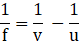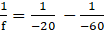### Solve the following examples. An object kept 60 cm from a lens gives a virtual image 20 cm in front of the lens. What is the focal length of the lens? Is it a converging lens or diverging lens?

Question 12.

Solve the following examples.

An object kept 60 cm from a lens gives a virtual image 20 cm in front of the lens. What is the focal length of the lens? Is it a converging lens or diverging lens?

Answer:

As per the question

The object distance is u=-60cm

Virtual image is v =-20cm⇒⇒ f= -30cm

From above we can conclude that as the focal length is negative it is diverging in nature and is a concave lens.